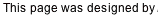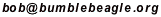You cannot run Java Applets with this browser!

This java applet computes the chance that a player will achieve a certain hitting streak at some point during the season. The model assumes that the chance that the player gets a hit in any game is unchanging and independent of other games.

You can specify the following:
• “Success Rate”— the probability that the player will get a hit in any given game.
• “Games In Season”— the total number of games the player will play in.
• “Streak Length”— the length of the hitting streak.
When you hit the “Compute” button, the applet will create a table showing the chance of such a streak at each point during the season. Note that longer streaks are also included. For example, if you ask for 56 game streaks, the results will also include 57 game streaks, 58, 59, and so on. When the computation is complete, you may wish to scroll the applet window up and down to view the entire table. You can also select the text and copy it into other applications.

The computation method is equivalent to the one used by Michael Frieman his article “56–Game Hitting Streaks Revisited” which appeared in The Baseball Research Journal, Number 31 (2003). Define z(n) = probability that such a streak has occurred within the first n games. If n is big enough (i.e. more than s), then upon the completion of game n, such an event (the event being that a streak of sufficient length has occurred) could have come about in two ways:
1. it happened in the first n-1 games
2. it has just happened for the first time in the nth game; in other words the streak has just reached length s
The probability of item (a) is z(n-1).

The probability of item (b) is 1-z(n-s-1) times 1-p times p^s. We had to have had no streak in the first n-s-1 games (1-z(n-s-1)), followed by a hitless game (1-p), followed by hits in s consecutive games (p^s).

Combining this with the simpler case for when n is less than s or equal to s, we arrive at this formula:
• when n < s, z(n) = 0
• when n = s, z(n) = p^s
• when n > s, z(n) = z(n-1) + (1-z(n-s-1)) * (1-p) * p^s
The applet shows a table of z(n) for the latter two cases.

Back to Bob’s main Baseball page.